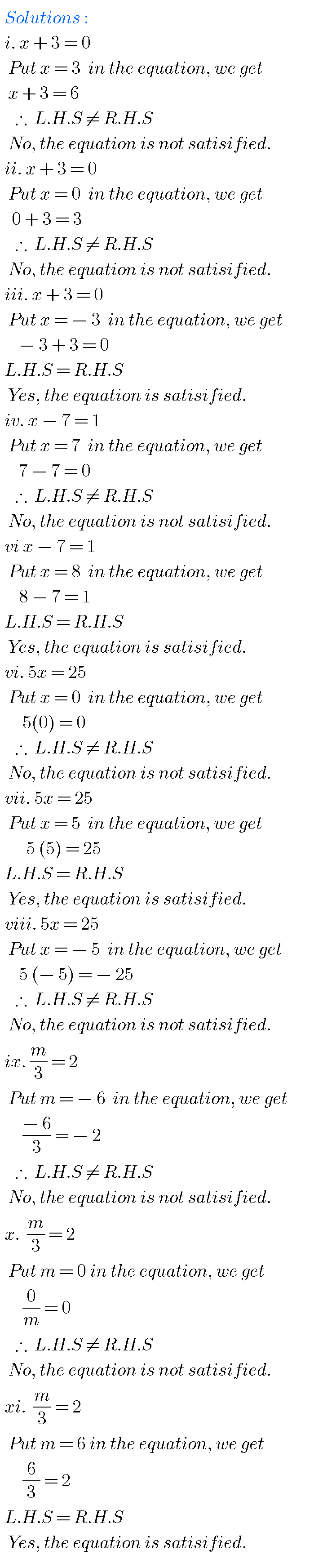# NCERT SOLUTIONS FOR CLASS 7 TH MATHS CHAPTER 4, SIMPLE EQUATIONS

Simple Equations class 7 chapter 4 maths Ncert solutions are given.

Study the textbook lesson Simple Equations very well.

Observe and Pracice the example problems and solutions given in the textbook.

Observe the given below solutions and try them in your own method.

You can see the solutions of some chapters for 7th class maths.

Chapter 1 Integers solutions 7 th class maths ncert

Chapter 2 Fractions and decimals solutions

7 th class maths ncert

Ncer solutions for 6 th class maths some chapters

Maths solutions class 10 real numbers

EXERCISE 4 . 1

#(adsbygoogle = window.adsbygoogle || []).push({});# PROBLEMS WITH SOLUTIONS FOR SIMPLE EQUATIONS CBSE

EXERCISE 4 . 2

M

EXERCISE 4 . 3

M

## PROBLEMS WITH SOLUTIONS FOR SIMPLE EQUATIONS CBSE

EXERCISE 4 . 4

M

Note : Observe the solutions of simple equations and try them in your own methods.

1. Integers

You can also see

Ncert solutions for maths class 6 some chapters

Ncert solutions for maths class 7 some chapters

Ncert solutions for maths class 8 some chapters

Intermediate maths solutions for some chapters

Ncert solutions for class 8 chapter 12

Nios solutions for maths 311 book 1 Sets 1.1